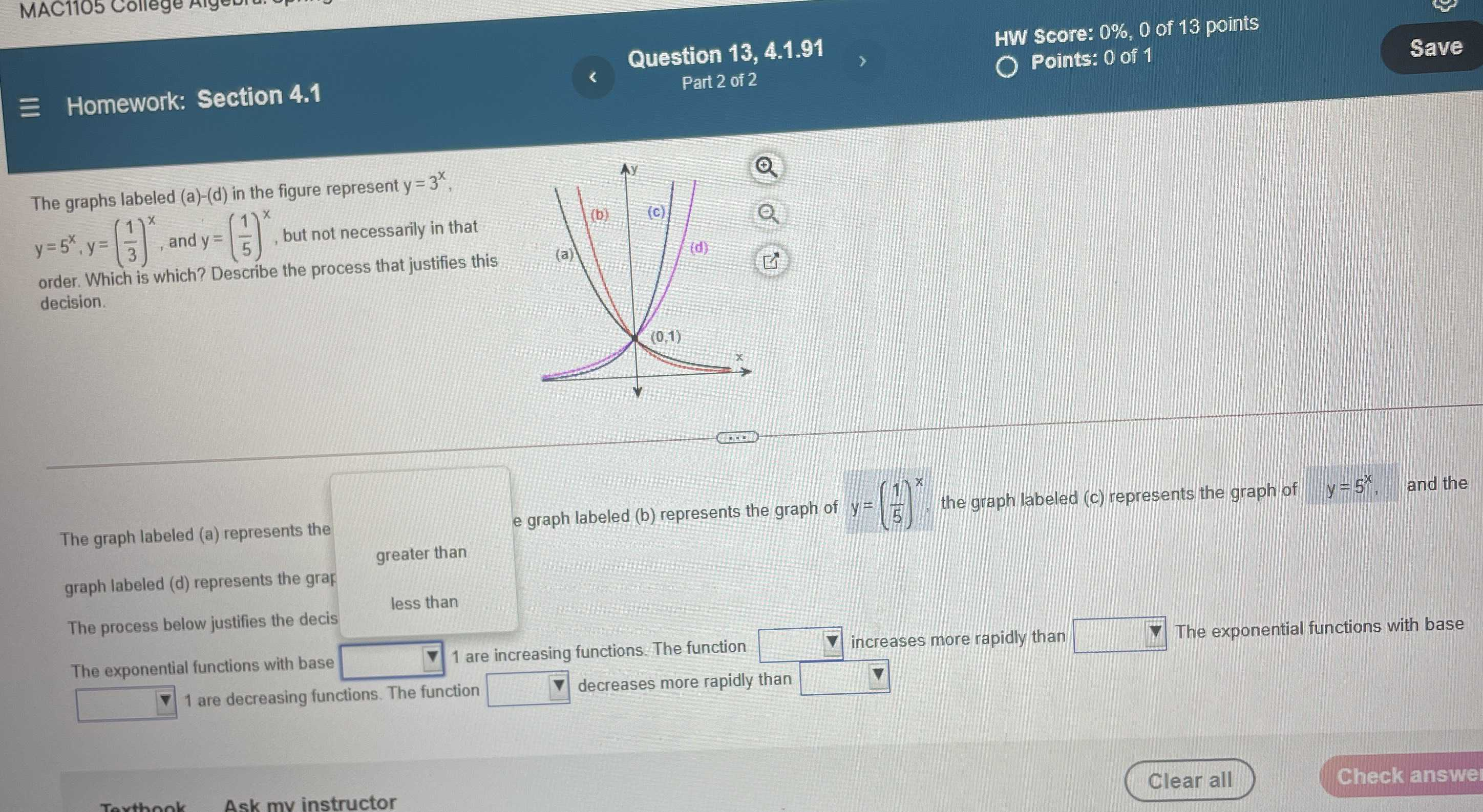### Still have math questions?

Algebra
QuestionThe graphs labeled (a)-(d) in the figure represent $$y = 3 ^ { x }$$ ,$$y = 5 ^ { x } , y = ( \frac { 1 } { 3 } ) ^ { x } ,$$ and $$y = ( \frac { 1 } { 5 } ) ^ { x }$$ , but not necessarily in that order. Which is which? Describe the process that justifies this decision. The graph labeled (a) represents the graph of $$y = ( \frac { 1 } { 3 } ) ^ { x }$$ , the graph labeled (b) represents the graph of $$y = ( \frac { 1 } { 5 } ) ^ { x }$$,the graph labeled (c) represents the graph of $$\quad y = 5 ^ { x } , \quad$$ and the graph labeled (d) represents the graph of $$y = 3 ^ { x }$$ .

The exponential functions with base $$\square 1$$ are increasing functions. The function $$\square$$increases more rapidly than $$\square$$ The exponential functions with base $$\square$$ 1 are decreasing functions. The function $$\square$$ decreases more rapidly than $$\square$$

$$y= 5^{x}$$         $$y= 3^{x}$$
$$y= (\frac{1}{5} )^x$$     $$y= (\frac{1}{3} )^x$$